#Gibbs PhenomenonOne shortcoming of Fourier series today known as the Gibbs phenomenon was first observed by H. Wilbraham in 1848 and then analyzed in detail by Josiah W. Gibbs (1839-1903). We will start with an example.

Example. Consider the function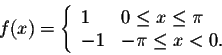Since this function is odd, we have an = 0, for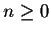. A direct calculation gives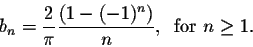for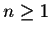. The Fourier partial sums of f(x) are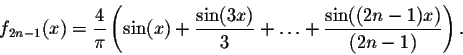The main Theorem implies that this sequence converges to f(x) except at the point x0 = 0, which a point of discontinuity of f(x). Gibbs got interested to the behavior of the sequence of Fourier partial sums around this point.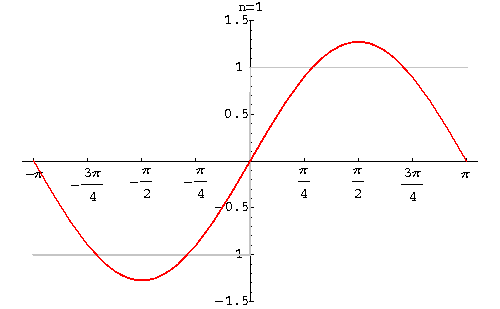Looking at the graphs of the partial sums, we see that a strange phenomenon is happening. Indeed, when x is close to the point 0, the graphs present a bump. Let us do some calculations to justify this phenomenon.
Consider the second derivative of f2n-1, which will help us find the maximum points.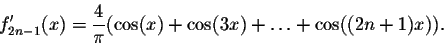Using trigonometric identities, we get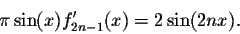So the critical points of f2n-1 are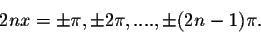Since the functions are odd, we will only focus on the behavior to the right of 0. The closest critical point to the right of 0 is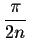. Hence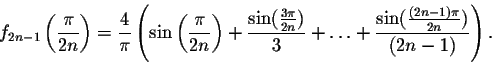In order to find the asymptotic behavior of the this sequence, when n is large, we will use the Riemann sums. Indeed, consider the function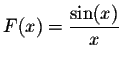on the interval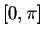, and the partition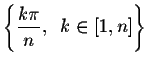of. So the Riemann sums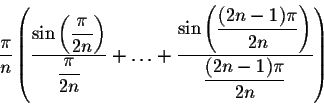converges to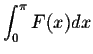. Esay calculations show that these sums are equal to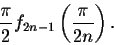Hence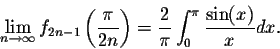Using Taylor polynomials of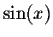at 0, we get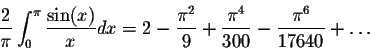i.e. up to two decimals, we have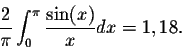These bumps seen around 0 are behaving like a wave with a height equal to 0,18. This is not the case only for this function. Indeed, Gibbs showed that if f(x) is piecewise smooth on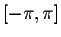, and x0 is a point of discontinuity, then the Fourier partial sums will exhibit the same behavior, with the bump's height almost equal to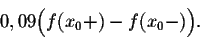To smooth this phenomenon, we introduce a new concept called the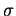-approximation. Indeed, let f(x) be a function piecewise smooth onand fN(x) its Fourier partial sums. Set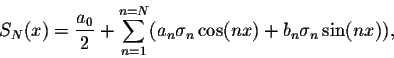wherewhich are called the-factors. To see that the sequence of sums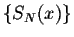better approximate the function f(x) than the Fourier partial sums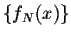, we use the following result:

Theorem. We have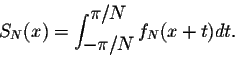Proof. We have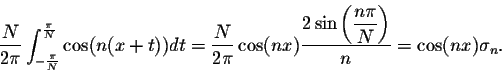Similarly, we have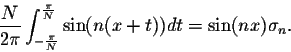Hence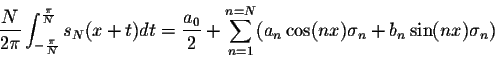which yields the conclusion above.

Using the above conclusion, we can easily see that indeed the sumsapproximate the function f(x) in a very smooth way. On the graphs, we can see that the Gibbs phenomenon has faded away.

Example. The picture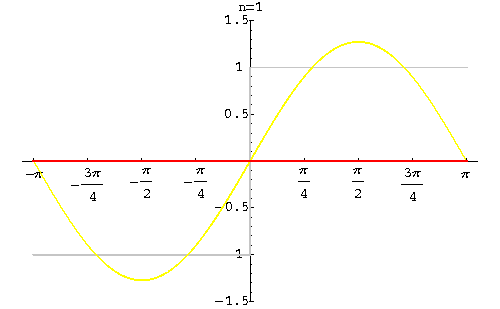shows how the-approximation helps fade away the Gibbs phenomenon for the function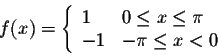Note that in this case, we have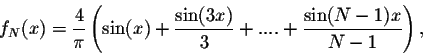and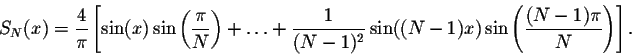[Geometry] [Algebra] [Trigonometry ]
[Calculus] [Differential Equations] [Matrix Algebra]S.O.S MATH: Home Page

Do you need more help? Please post your question on our S.O.S. Mathematics CyberBoard.Author: M.A. Khamsi